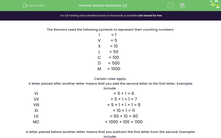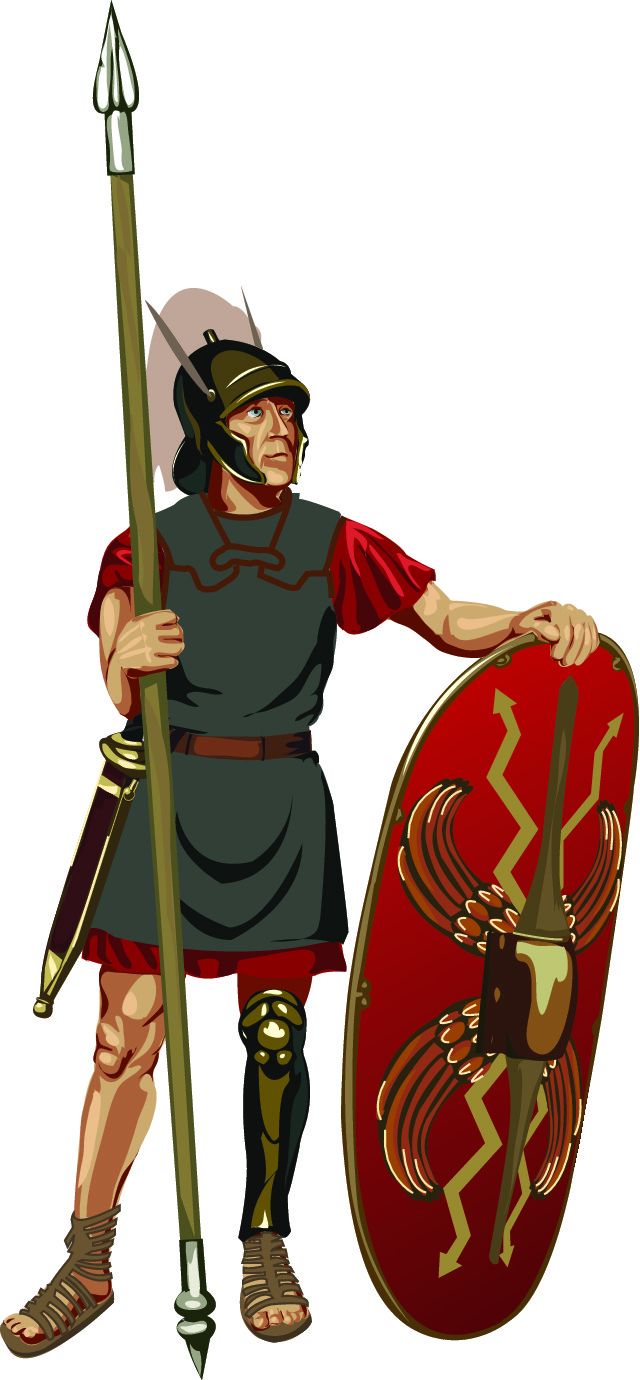# Understand Roman Numerals (2)

In this worksheet, students will convert from normal numbers to Roman numerals.Key stage:  KS 2

Curriculum topic:   Number: Number and Place Value

Curriculum subtopic:   Read Roman Numerals to M (1000)

Difficulty level:#### Worksheet Overview

The Romans used the following symbols to represent their counting numbers:

 I = 1 V = 5 X = 10 L = 50 C = 100 D = 500 M = 1000

Certain rules apply.

A letter placed after another letter means that you add the second letter to the first letter. Examples include:

 VI = 5 + 1 = 6 VII = 5 + 1 + 1 = 7 VIII = 5 + 1 + 1 + 1 = 8 XI = 10 + 1 = 11 LX = 50 + 10 = 60 MC = 1000 + 100 = 1100

A letter placed before another letter means that you subtract the first letter from the second. Examples include:

 IV = 5 - 1 = 4 IX = 10 - 1 = 9 XL = 50 - 10 = 40 CM = 1000 - 100 = 900

Example

Write the number 3874 in Roman numerals.

MMM = 3000

DCCC = 500 + 300 = 800

L = 50

XX = 20

IV = 5 - 1 = 4

So 3874 = MMMDCCCLXXIV

Phew, that's quite a number! Are you ready to have a go at these now?### What is EdPlace?

We're your National Curriculum aligned online education content provider helping each child succeed in English, maths and science from year 1 to GCSE. With an EdPlace account you’ll be able to track and measure progress, helping each child achieve their best. We build confidence and attainment by personalising each child’s learning at a level that suits them.

Get started